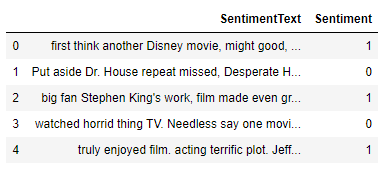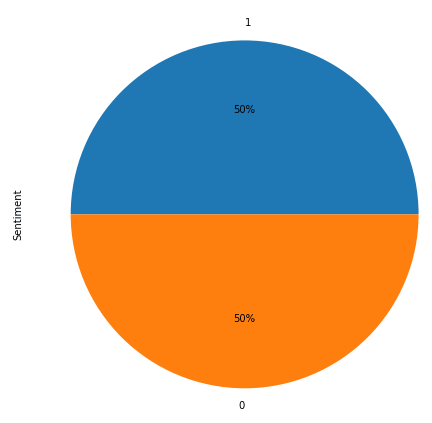Text sentiment analysis refers to the process of finding the sentiment from public opinions in the form of text. Sentiment analysis has various applications. For instance, organizations need to know what people think about their products, especialy whether they like or dislike their products. The products that are liked should be produced in higher number and the products disliked should be improved.

Manually detecting sentiment from thousands of text documents or emails can be time consuming and costly. With the advent of machine learning techniques, finding public sentiment from text has become simpler, faster and easier.

In this tutorial, we’ll demonstrate how we can use Python to analyze sentiment from public reviews for different movies. We’ll use Python’s scikit-learn library for machine learning. You’ll often see scikit-learn referred to as sklearn. We’ll use the same convention in this tutorial.

## The Dataset

The dataset for this tutorial can be downloaded freely from this Kaggle link. Download the CSV file to your local system. We’re going to use this dataset to train our machine learning program so we can see how it does analyzing sentiments.

The next step is to import the libraries that we will be using in this tutorial along with the dataset.

The following script imports the required libraries:

``````import numpy as np
import re
import nltk
import pandas as pd
from nltk.corpus import stopwords
import matplotlib.pyplot as plt
%matplotlib inline``````

To import the dataset, we will make use of the read_csv method of the Pandas library. The following script imports the data and prints the first five rows of the data using the `head()` method. Simply update the file path to match the location of your csv file if it’s in a different folder than your python script.

``````imdb_reviews = pd.read_csv(r"E:/Datasets/imdb_reviews.csv", encoding = "ISO-8859-1")

Output:You can see that the dataset has two columns: SentimentText and Sentiment. The column names are quite self-explanatory. The first column contains sentiment text, while the second column contains sentiment in the form of binary values. Here 1s refers to positive sentiments while 0s refers to negative sentiments.

I put together a Python Developer Kit with over 100 pre-built Python scripts covering data structures, Pandas, NumPy, Seaborn, machine learning, file processing, web scraping and a whole lot more - and I want you to have it for free. Enter your email address below and I'll send a copy your way.

Yes, I'll take a free Python Developer Kit

Let’s view the distribution of positive and negative sentiment:

``````plt.rcParams["figure.figsize"] = [10, 8]
imdb_reviews.Sentiment.value_counts().plot(kind='pie', autopct='%1.0f%%')``````

Output:The output shows that half of the reviews have positive sentiments while the remaining reviews contain negative sentiments.

## Data Preprocessing

Before we can use machine learning models from the scikit-learn library to perform sentiment analysis, we need to process our data and convert it to a format that can be used as input to scikit-learn machine learning models.

The first preprocessing step is to divide the data into features and labels sets. In machine learning models, a feature set is used to predict the labels. In other words, machine learning models try to find a statistical relationship between the input features and output labels. Our feature set will contain sentiment text while the labels will contain actual sentiments.

``````reviews = imdb_reviews["SentimentText"].tolist()
labels = imdb_reviews["Sentiment"].values``````

Here, the reviews list contains text reviews and the labels variable contains corresponding sentiments. The text reviews we’re using contain several special characters and are in upper and lower cases. We need to clean our text by removing all the special characters and then converting all the text to lower case for the sake of uniformity. We can use Python’s regular expressions for this purpose as shown in the following script:

``````processed_reviews = []

for text in range(0, len(reviews)):

text = re.sub(r'\W', ' ', str(reviews[text]))

text = re.sub(r'\s+[a-zA-Z]\s+', ' ', text)

text = re.sub(r'\^[a-zA-Z]\s+', ' ', text)

text = re.sub(r'\s+', ' ', text, flags=re.I)

text = re.sub(r'^b\s+', '', text)

processed_reviews.append(text)``````

Once text has been cleaned, the next step is to divide the dataset into training and test sets. The training set is used to train a machine learning model, while the test set is used to evaluate the performance of a machine learning model. We will divide our data into an 80% training set and a 20% test set. We can use the `train_test_split()` function from the scikit-learn library to easily divide our data into training and test sets:

``````from sklearn.model_selection import train_test_split
X_train, X_test, y_train, y_test = train_test_split(processed_reviews, labels, test_size=0.2, random_state=0)``````

## Converting Text to Numeric Form

Machine learning models are based on statistical and mathematical algorithms and mathematical algorithms work with numbers. Our feature set consists of text-based sentences. There are several ways to convert text to numeric form. Some of the most common techniques are bag of words model, TF-IDF model, [word embeddings] and (https://towardsdatascience.com/what-the-heck-is-word-embedding-b30f67f01c81), transform models.

For the sake of simplicity, we’ll be using TF-IDF model since it’s natively compatible with scikit-learn. Sklearn makes the TF-IDF model easy to implement, but it’s actually quite advanced. For more advanced deep learning techniques, you can use word embeddings or transform models.

The `TfidfVectorizer` class of sklearn lets you convert text to TF-IDF compliant numeric form. The following script converts both the training and test sentences to TF-IDF numeric vectors:

``````from sklearn.feature_extraction.text import TfidfVectorizer
vectorizer = TfidfVectorizer(max_features=2000, min_df=5, max_df=0.75, stop_words=stopwords.words('english'))
X_train = vectorizer.fit_transform(X_train).toarray()
X_test = vectorizer.transform(X_test).toarray()``````

## Training the Algorithm

The scikit-learn library contains various algorithms that can be used to classify text sentiment. We’ll be using the Random Forest algorithm which is one of the most commonly used machine learning algorithms because of its excellent accuracy. To train the model, you need to pass the training features and labels to the `fit()` method of the `RandomForestClassifier` class. Run the following script to train the model.

``````from sklearn.ensemble import RandomForestClassifier
rfc = RandomForestClassifier(n_estimators=200, random_state=42)
rfc.fit(X_train, y_train)``````

Once the model is trained, we need to know how good our model is. To do so, we can make predictions on the test set and then compare the predicted sentiments with the actual sentiments to find the accuracy of the trained model. To make predictions on our test set, you need to pass the test set to the `predict()` method of the object that contains the trained algorithm.

``y_pred = rfc.predict(X_test)``

Finally, to find the accuracy of predictions, you can use the `accuracy_score` method of the `sklearn.metrics` module.

``````from sklearn.metrics import  accuracy_score
print(accuracy_score(y_test, y_pred))``````

Output: 0.8466

The output shows an accuracy of 84.66% which is pretty impressive.

To make creating your own sklearn sentiment analysis code even easier, here is the complete Python code for the application developed in this tutorial:

``````import numpy as np
import re
import nltk
import pandas as pd
from nltk.corpus import stopwords
import matplotlib.pyplot as plt
%matplotlib inline

imdb_reviews = pd.read_csv(r"E:/Datasets/imdb_reviews.csv", encoding = "ISO-8859-1")

plt.rcParams["figure.figsize"] = [10, 8]
imdb_reviews.Sentiment.value_counts().plot(kind='pie', autopct='%1.0f%%')

reviews = imdb_reviews["SentimentText"].tolist()
labels = imdb_reviews["Sentiment"].values

processed_reviews = []

for text in range(0, len(reviews)):
text = re.sub(r'\W', ' ', str(reviews[text]))

text = re.sub(r'\s+[a-zA-Z]\s+', ' ', text)

text = re.sub(r'\^[a-zA-Z]\s+', ' ', text)

text = re.sub(r'\s+', ' ', text, flags=re.I)

text = re.sub(r'^b\s+', '', text)

processed_reviews.append(text)

from sklearn.model_selection import train_test_split
X_train, X_test, y_train, y_test = train_test_split(processed_reviews, labels, test_size=0.2, random_state=0)

from sklearn.feature_extraction.text import TfidfVectorizer
vectorizer = TfidfVectorizer(max_features=2000, min_df=5, max_df=0.75, stop_words=stopwords.words('english'))
X_train = vectorizer.fit_transform(X_train).toarray()
X_test = vectorizer.transform(X_test).toarray()

from sklearn.ensemble import RandomForestClassifier

rfc = RandomForestClassifier(n_estimators=200, random_state=42)
rfc.fit(X_train, y_train)
y_pred = rfc.predict(X_test)

from sklearn.metrics import classification_report, confusion_matrix, accuracy_score

print(confusion_matrix(y_test,y_pred))
print(classification_report(y_test,y_pred))
print(accuracy_score(y_test, y_pred))``````

## Conclusion

Text sentiment analysis is a very important task in natural language processing. In this tutorial, you saw how we can use the scikit-learn library to perform text sentiment analysis in Python.

For more ways you can use Python for machine learning, please subscribe using the form below. We’ll send you some helpful tutorials to make sure you’re getting the most out of Python.